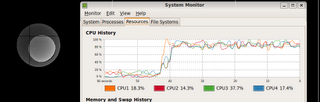## Sunday, 4 January 2009

### Ray Tracing in Clojure (Part II)

In my previous post, I ported across a ray-tracing example from ANSI Common Lisp. Now, I'll try and work out how threading functions work.

Each area of the viewing image is independent, so let's divide up the viewing surface into a number of independent buffered images. We have a little helper function which given a width, height and tile size gives us back a list of positions to draw at.

```(defn create-work-list [width height unitX unitY]
(let [xs (range 0 width unitX) ys (range 0 height unitY)]
(mapcat (fn [x] (mapcat (fn [y] (list (list x y))) ys)) xs)))
```

For example, if we want to draw use a tile size of 150x150 with a viewing surface of 300x300 we get the following offsets:

```user> (create-work-list 300 300 150 150)
((0 0) (0 150) (150 0) (150 150))
```

Now we should make the `ray-trace` function take notice of these co-ordinates. The previous version of the ray-trace function wasn't very functional as it performed IO (drawing to the canvas). Side-effects are the enemy of referential transparency.

```(defn ray-trace [world w h ox oy]
(let [buffered-image (BufferedImage. w h BufferedImage/TYPE_BYTE_GRAY)]
(doseq [x (range 0 (dec w))]
(doseq [y (range 0 (dec h))]
(.setRGB buffered-image x y (color-at (+ x ox) (+ y oy)))))
buffered-image))
```

Now the function is pure because it always gives the same output for the same input and no IO occurs.

Finally, we need to distribute this work across all the cores. Clojure has a built in function, pmap that works the same as map but in parallel.

Initially I performed the drawing to the canvas within a `pmap` expression - this is very wrong! `pmap` is lazy, it's not evaluated unless it is needed. Clojure doesn't know that I intended that to always been evaluated, so it was only ever drawing the first four tiles (presumably because that's how many cores my desktop has).

If you want to force the evaluation there's a number of functions which do that:
• `doseq`
• `dorun`
• `doall`
In this case I decided I'd use `ray-trace` in a `pmap` expression to produce a list of images, and then use `doseq` to perform the side effects.
```(def canvas (proxy [JPanel] []
(paintComponent [g]
(proxy-super paintComponent g)
(.setColor g Color/RED)
(let [width (.getWidth this) height (.getHeight this) unitX (/ width 16) unitY (/ height 16) work-list (create-work-list width height unitX unitY)]
(doseq [image (pmap (fn [pos] (list (apply ray-trace (list world unitX unitY (first pos) (second pos))) (first pos) (second pos))) work-list)]
(.drawImage g (first image) (second image) (nth image 2) unitX unitY nil))))))
```
The separation of IO and pure functions is something advocated in "A wish list for the next mainstream programming language". Clojure doesn't force this, whereas something like Haskell does. Haskell uses monads to achieve this, which is something I'll visit at some point. See LtU for some explanations. Ok, enough theory - what difference does this actually make? Well for me, about a 4x difference, and I have 4 cores, so that's good! Timing's aren't that exciting, but you can see the difference with the system monitor.Pretty much full CPU utilization across all cores, with only a few lines of code changed, funky!

1.Hi there. I have also written a ray tracer in Clojure as a learning exercise. You can see sample images and download the source from http://tu.be/graphics

In case you are interested, here are some techniques for numerical performance improvement in Clojure code. These techniques gave my ray tracer a 25-30% speed boost over storing data in structmaps/vectors, and a 5-20x boost for the routines themselves.

1. Use double-array or float-array to store the data (points, vectors, etc).
2. Use aset to store the data in the arrays (faster than aset-double/float). Write macros that use aget to access to the data.
3. Use (double) or (float) to cast values that will be put in a vector into that type (speeds creation of new vectors, allows use of aset instead of aset-double).
4. Use type hints for any routine that takes arrays of primitives as arguments e.g. (defn vec-op [#^floats vec1 #^floats vec2] ... )
5. Use nested arithmetic e.g. (+ a (+ b c)) instead of (+ a b c)

(There are most likely other tricks, like using mutable data to reduce the number of object creations).

Using those techniques together avoids unboxing/boxing overhead, reduces vector/point/etc creation time, and allows the use of primitive arithmetic. This should get you about the same speed as writing the code in Java.

I also recommend using a profiler, as the bottlenecks are often in non-intuitive places. For example, I found that using macros for functions like dot-product sometimes reduced performance. This may be by making the code larger and less able to fit in the CPU cache, or because the Java runtime can better optimize performance if the code is isolated in one function that is called many times, or some other reason...

For multi-threading, I used agents, but I think the results are about the same.

These are just my findings so far, and I am interested to learn any improvements on these techniques.

2.That looks really cool! I got a 404 when I tried to download the source though?

I definitely didn't have performance in mind when I wrote this code (it was more or less a literal translation from a book).

Great tips on performance optimization too so thanks for those!

I've looked at using JVisualVM for profiling, it's really good for seeing what's taking the time.

jeff

3.4.